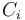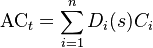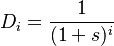## Definition

Credit-Adjusted Effective Interest Rate, in the context of IFRS 9 , is the interest rate that exactly discounts estimated future cash payments or receipts through the expected life of the financial asset to the amortised cost of a financial asset that is a purchased or originated credit-impaired financial asset.

## Calculation

When calculating the credit-adjusted effective interest rate, an entity shall estimate the expected cash flows by considering all contractual terms of the financial asset (for example, prepayment, extension, call and similar options) and expected credit losses.

The calculation includes all fees and points paid or received between parties to the contract that are an integral part of the effective interest rate (see paragraphs B5.4.1‒B5.4.3 of IFRS 9), transaction costs, and all other premiums or discounts.

There is a presumption that the cash flows and the expected life of a group of similar financial instruments can be estimated reliably. However, in those rare cases when it is not possible to reliably estimate the cash flows or the remaining life of a financial instrument (or group of financial instruments), the entity shall use the contractual cash flows over the full contractual term of the financial instrument (or group of financial instruments).

## Formula

Given a set of expected${\textstyle C_{i}}$ (with index running from 1 to n) and a set of discount factors D calculated using the credit-adjusted effective interest rate (s) over the expected life T of a financial asset, that rate is derived by solving the equation$\mbox{AC}_t = \sum_{i=1}^{n} D_{i}(s) C_{i}$

where the discount factor for period (i) is given by$D_i = \frac{1}{(1 + s)^{i}}$

The formula for the credit-adjusted effective interest rate is identical with the calculation of the Effective Interest Rate. The two key differences are:

• the discounted cashflows must match the amortised cost, not the gross carrying amount (which is net of any Loss Allowance
• the expected cashflows must be adjusted for expected credit losses